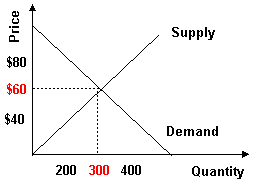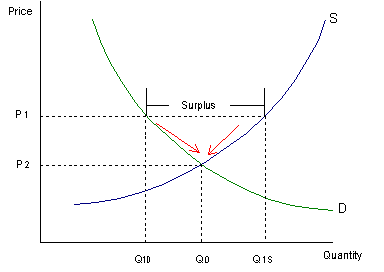### Seeing is believing!

Before you order, simply sign up for a free user account and in seconds you'll be experiencing the best in CFA exam preparation.

### Subject 3. Market Equilibrium

Aggregate Demand and Aggregate Supply

An aggregate demand curve is simply a schedule that shows amounts of a product that buyers collectively desire to purchase at each possible price level.

An aggregate supply curve is simply a curve showing the amounts of a product that all firms will produce at each price level.

Example 1

Refer to the graph below. What is the market quantity that would be supplied at a price of \$2.00?Market quantity is the sum of individual quantities supplied at each price. At a price of \$2.00, Ann supplies 4, Barry supplies 3, and Charlie supplies 0. The market supply is 7.

Market Equilibrium

Equilibrium is a state in which conflicting forces are in balance. In equilibrium, it will be possible for both buyers and sellers to realize their goals simultaneously.

The following graph depicts the market supply and demand for concert tickets at Madison Square Garden in New York City.Equilibrium price and quantity are where the supply and demand curves intersect. Draw a horizontal line from the intersection to the price axis. This is equilibrium price: \$60. Draw a vertical line from the intersection to the quantity axis. This is equilibrium quantity: 300. It is equilibrium because quantity demanded equals quantity supplied at \$60 per ticket. At this price, there is neither surplus (excess supply) nor shortage (excess demand), so there is no downward or upward pressure for the price to change.

Surplus will push prices downward towards equilibrium.• Suppose the price is initially above the equilibrium price (P2) and sits at P1.
• Quantity supplied (Q1s) will exceed quantity demanded (Q1D), creating a surplus.
• The surplus will put downward pressure on prices since producers will begin to lower their prices to sell the surplus.
• As a result, the price will fall, the quantity supplied will decrease, and the quantity demanded will increase until the equilibrium price (P2) is restored.
• This process involves movements along supply-and-demand curves since the changes are caused by price fluctuations.

Similarly, shortages push prices upward towards equilibrium.

Because the price rises if it is below equilibrium, falls if it is above equilibrium, and remains constant if it is at equilibrium, the price is pulled toward equilibrium and remains there until some event changes the equilibrium. We refer to such an equilibrium as being stable because whenever price is disturbed away from the equilibrium, it tends to converge back to that equilibrium.

An unstable equilibrium is an equilibrium that is not restored if disrupted by an external force. While most equilibria studied in economics are of the stable variety, a few cases of unstable equilibria do emerge from time to time, in limited circumstances.

#### Practice Question 1

Price tends to be in equilibrium where supply and demand intersect because ______

A. when quantity supplied equals quantity demanded, prices don't change.
B. when quantity supplied exceeds quantity demanded, prices have a tendency to rise.
C. when quantity supplied is less than quantity demanded, prices tend to fall.

When quantity supplied equals quantity demanded, prices don't change. When prices are not changing, the market is said to be in equilibrium.

#### Practice Question 2

Suppose excess demand causes prices to rise. As prices rise, ______

A. the quantity demanded and supplied will rise.
B. the quantity demanded will rise and the quantity supplied will fall.
C. the quantity demanded will fall and the quantity supplied will rise.

This is an application of the law of demand and the law of supply.

#### Practice Question 3

In the 1990s, the 76 million U.S. baby boomers began to put away more and more savings for retirement. What is the effect of this event on the equilibrium price and quantity of financial assets?

A. Price rises and quantity also rises.
B. Price rises and quantity falls.
C. Price falls and quantity rises.

The saving of the U.S. baby boomers increases the demand for financial assets, increasing the equilibrium price and quantity.

#### Practice Question 4

A Wall Street Journal headline reads: "Cigar Shortage Draws New Brands into Market." The shortage resulted from a renewed interest in smoking cigars. What best describes facts behind the headline?

A. Supply has shifted to the right. Price has fallen somewhat, but not enough to equilibrate supply and demand.
B. Demand has shifted to the right. Price has risen somewhat, increasing quantity supplied but not enough to equal quantity demanded.
C. Demand has shifted to the right and price has risen to equilibrate supply and demand.

The renewed interest in cigars shifted the demand for cigars to the right. Since a shortage of cigars still exists, price has not risen enough to equilibrate supply and demand. There is no evidence of declining supply.

#### Practice Question 5

Market equilibrium exists when ______

I. there is no government intervention in the market.
II. the demand curve intersects the quantity axis.
III. the supply curve intersects the price axis.
IV. the quantity demanded equals the quantity supplied.

In equilibrium, quantity demanded equals quantity supplied. This occurs where the supply and demand curves intersect.

#### Practice Question 6

If the supply of a product decreases and the demand for that product simultaneously increases, then ______

A. equilibrium price must rise, but equilibrium quantity may either rise, fall, or remain unchanged.
B. equilibrium price must rise and equilibrium quantity must fall.
C. equilibrium price and equilibrium quantity must both increase.

#### Practice Question 7

You observe that the price of bread has increased. From this, you can conclude that ______

A. demand has increased.
B. supply has increased.
C. demand has decreased.
D. you would need further information on quantity changes in order to come to a conclusion.

An increase in price could mean either that supply has decreased or that demand has increased, but without information on whether the equilibrium quantity has risen or fallen, it is impossible to tell which has occurred.

#### Practice Question 8

The market demand curve will always ______

A. be flatter than the individual demand curves that make it up.
B. be steeper than the individual demand curves that make it up.
C. have the same slope as the individual demand curves that make it up.

An individual firm's demand curve is always horizontal in a competitive market.

#### Practice Question 9

When a market is in long-run equilibrium, ______

A. the opportunity cost of producing a good equals the market price of the good.
B. the price elasticity of demand for the good equals the price elasticity of supply for the good.
C. the quantity demanded exceeds the quantity supplied.

In order for long-run equilibrium to hold, the amount supplied and the amount demanded in the market must be equal; additionally, the opportunity cost of producing the product must equal the market price.

#### Practice Question 10

Suppose that initially the equations for demand and supply are Qd = 48 - 4P and Qs = 4P - 16, respectively. If the quantity supplied decreases by 4 at every price (so that the supply curve shifts to the left), the equilibrium price will change from ______.

A. \$8 to \$7.50
B. \$8 to \$8.50
C. \$8.50 to \$7.50

To solve for equilibrium price, set Qd equal to Qs. The initial equilibrium price is \$8. The equation for the new supply curve is Qs = 4P -20 and the new equilibrium price is 8.5.

#### Practice Question 11

If the supply of a good decreases, which of the following will generally occur in a market setting?

A. The quantity demanded will decrease.
B. The quantity demanded will increase.
C. Demand will decrease.

An inward shift of the supply curve for a good implies that equilibrium will occur at a higher price and lower output. This is because the demand curve (which remains constant) and the supply curve now intersect at a higher price and lower output.

#### Practice Question 12

The market demand for an item is ______.

A. the vertical summation of individual demand curves
B. the horizontal summation of individual demand curves
C. smaller than the sum of individual demands because of competition for the same units